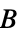AtomicDensityMatrix`
AtomicDensityMatrix`

# AtomicState

AtomicState[label]

represents a labeled atomic state.

AtomicState[label,par1val,par2val,]

represents an atomic state with one or more specified atomic parameters

# Details and Options

• An atomic system is the first argument to many of the functions in the AtomicDensityMatrix package.
• The label can be a number, string, or other expression. However, use of expressions other than numbers or strings as labels can reduce functionality when forming state selection criteria in functions like SelectStates.
• The following atomic parameters can be specified:
•  L electronic orbital-angular-momentum quantum number S electronic spin-angular-momentum quantum number J total electronic angular-momentum quantum number NuclearSpin nuclear angular-momentum quantum number F total angular-momentum quantum number M-projection of total angular momentum Parity parity; either Even or Odd Energy unperturbed energy (not including hyperfine shift in a hyperfine system) GFactor-factor NaturalWidth natural linewidth Polarizability[rank] electric polarizability (scalar, vector or tensor) HyperfineA hyperfinecoefficient HyperfineB hyperfinecoefficient BranchingRatio[lab] branching ratio to level with label lab
• A list of AtomicState objects is referred to as an atomic system.

# Examples

## Basic Examples(3)

Atomic system consisting of two AtomicState objects.

Atomic system with Zeeman structure. Sublevels is used to generate the Zeeman sublevels of the two specified states.

Atomic system with hyperfine structure: